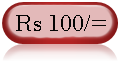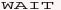Web Development : Starting from Concept, HTML, CSS, Web Site Develpment to Website Launching.
Written by: Sanjay Srivastava
B.Tech (Honours);IIT Kharagpur
PMP ; PMI USA

Class - VIII - Maths:- INDEX

1. Squares & Square Roots
2. Cubes & Cube Roots
4. Profit Loss & Discount
5. Compound Interest
6. Algebraic Identities7. Polynomials8. Linear Equations9. Parallel Lines10. Types of Quadrilaterals11. Construction of Quadrilaterals12. Circles13. Areas14. Surface Areas15. Volumes16. StatisticsClass - VIII - Maths

## What is Compound Interest Formula (Page 2/3)

Important Tips (1) Be careful to read during the exam regarding the formula of simple interest or compound interest.
(2) If the interest rate is doubled / tripled, then the simple interest rate will get doubled / tripled. In case of compound interest the increase will be more than the increase of rate of interest

Question - Type 2 - What is the loss in interest if Rs 20000 deposited for 3 years at interest rate of 10% per annum and simple interest is calculated in place of compound interest.

Answer - 2- Simple Interest = 20000 * 3 * 10 /100
20000 * .3 = Rs 6000

Compound Interest = 20000 *((1+10/100)^3-1)
= 20000 * ((1.1)^3-1)) = 20000 (.331) = Rs 6620
Therefore loss = C.I. - S.I.
= 6620 - 6000 = Rs 620

Question - Type 3 - If the interest rate in the previous questions is doubled. Will interest will be doubled in both the cases i.e. simple and compound interest.

Answer - 3- YES & NO - In case of simple interest the interest amount will be doubled. But in the case of compound interest, it will be more than double. (refer next question on next page.)